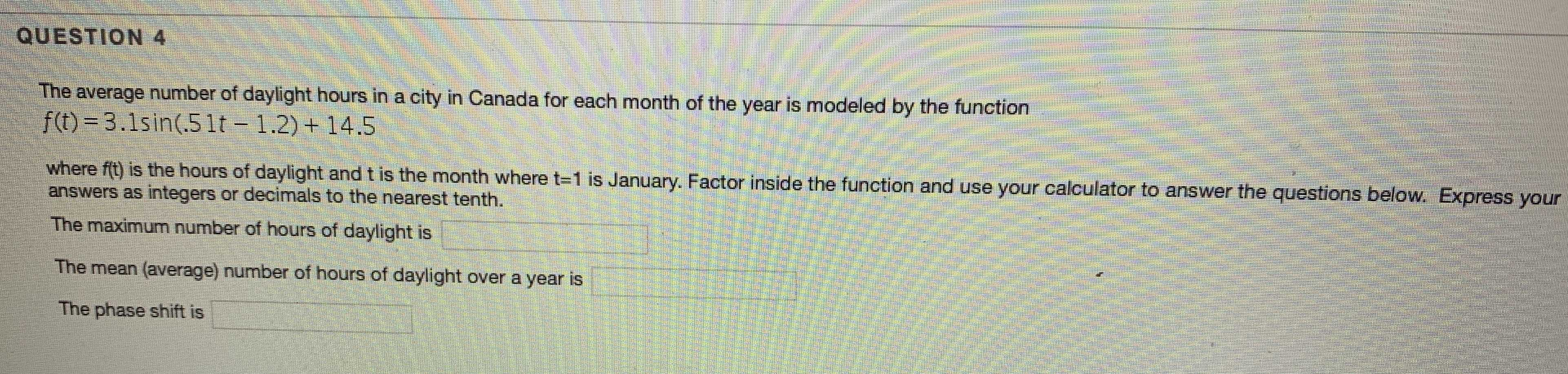### Still have math questions?

Algebra
QuestionQUESTION $$4$$ The average number of daylight hours in a city in Canada for each month of the year is modeled by the function

$$f ( t ) = 3.1 \sin ( .51 t - 1.2 ) + 14.5$$

where $$f ( t )$$ is the hours of daylight and $$t$$ is the month where $$t = 1$$ is January. Factor inside the function and use your calculator to answer the questions below. Express your answers as integers or decimals to the nearest tenth. The maximum number of hours of daylight is The mean (average) number of hours of daylight over a year is The phase shift is